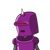# 17. Using the identity a – b = (a + b) (a -b), find the value of (2x + y) – (2x – y).​

17. Using the identity a – b = (a + b) (a -b), find the value of (2x + y) – (2x – y).​

### 1 thought on “17. Using the identity a – b = (a + b) (a -b), find the value of (2x + y) – (2x – y).​”

1.Step-by-step explanation:

=(2x+y)-(2x-y)

=2x+y-2x+y

=2y

This identity a-b=(a+b)(a-b)

bcause you used negative sign to subtract